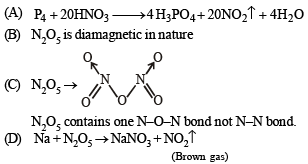Courses

# Test: MCQs (One or More Correct Option): The p-Block Elements | JEE Advanced

## 19 Questions MCQ Test Class 12 Chemistry 35 Years JEE Mains &Advance Past yr Paper | Test: MCQs (One or More Correct Option): The p-Block Elements | JEE Advanced

Description
This mock test of Test: MCQs (One or More Correct Option): The p-Block Elements | JEE Advanced for JEE helps you for every JEE entrance exam. This contains 19 Multiple Choice Questions for JEE Test: MCQs (One or More Correct Option): The p-Block Elements | JEE Advanced (mcq) to study with solutions a complete question bank. The solved questions answers in this Test: MCQs (One or More Correct Option): The p-Block Elements | JEE Advanced quiz give you a good mix of easy questions and tough questions. JEE students definitely take this Test: MCQs (One or More Correct Option): The p-Block Elements | JEE Advanced exercise for a better result in the exam. You can find other Test: MCQs (One or More Correct Option): The p-Block Elements | JEE Advanced extra questions, long questions & short questions for JEE on EduRev as well by searching above.
*Multiple options can be correct
QUESTION: 1

### In the electrolysis of alumina, cryolite is added to :

Solution:

Because of high melting point (2050ºC), pure alumina cannot be electrolysed. Hence a mixture of alumina, cryolite (m.p. 1000ºC) and calcium fluoride (to lower the temperature of the melt) is electrolysed at about 900ºC.
NOTE : The function of cryolite is to increase the electrical conductivity of the electrolyte, and to lower the temperature of the melt.

*Multiple options can be correct
QUESTION: 2

### Nitrogen(I) oxide is produced by :

Solution:

NH4NO3 ——→ N2O + 2H2O
NH2OH.HCl + NaNO2 ——→ N2O + NaCl + 2H2O

*Multiple options can be correct
QUESTION: 3

### The compounds used as refrigerant are

Solution:

NH3 and CF2Cl2 (freon-12) are used as refrigerants.

*Multiple options can be correct
QUESTION: 4

The major role of fluorspar (CaF2), which is added in small quantities in the electrolytic reduction of alumina dissolved in fused cryolite (Na3AlF6), is

Solution:

To make the fused mixture very conducting and to reduce the temperature of the melt.

*Multiple options can be correct
QUESTION: 5

The material used in the solar cells contains

Solution:

Silicon is used in solar cells.

*Multiple options can be correct
QUESTION: 6

Sodium nitrate decomposes above  800°C  to give

Solution:

Sodium nitrate on decomposition upto 500oC gives NaNO2 and oxygen.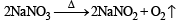While at higher temperature (i.e. above to 800°C), NaNO2 further decomposes into  Na2O, N2 and O2.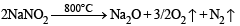*Multiple options can be correct
QUESTION: 7

White phosphorus (P4) has

Solution:

The four atoms in a P4 molecule are situated at the corners of a tetrahedron. There are six P - P single bonds with PPP bond angle equal to 60o. Each phosphorus has a lone pair of electrons.

*Multiple options can be correct
QUESTION: 8

Ammonia, on reaction with hypochlorite anion, can form

Solution:

2NH3 + OCl–→ NH2.NH2 + H2O + Cl

*Multiple options can be correct
QUESTION: 9

A solution of colourless salt H on boiling with excess NaOH produces a non-flammable gas. The gas evolution ceases after sometime. Upon addition of Zn dust to the same solution, the gas evolution restarts. The colourless salt (s) H is (are)

Solution:

When ammonium salt NH4NO3 or NH4NO2 (ammonium salts are colourless) is boiled with excess of NaOH, ammonia (NH3) gas is evolved as follows:

NH4NO2 + NaOH → NaNO2 + NH3 + H2O
NH4NO3 + NaOH → NaNO3 + NH3 + H2O

The NH3 gas evolved is non-flammable gas.
When the gas evolution ceases we are left with NaNO2 or NaNO3 in solution.
These salts get reduced when Zn is added to this solution containing salt (NaNO2 or NaNO3) and excess NaOH and NH3 gas is evolve.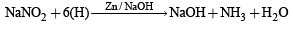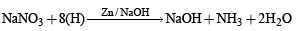Thus the colourless salt [H] is either NH4NO2 or NH4NO3.
Thus (a) and (b) are correct answers. [NOTE : NaCl formed has no reaction with NaOH]

*Multiple options can be correct
QUESTION: 10

The nitrogen oxide(s) that contain(s) N-N bond(s) is(are)

Solution: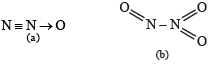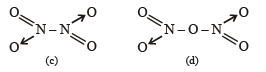*Multiple options can be correct
QUESTION: 11

Which of the following halides react(s) with AgNO3(aq) to give a precipitate that dissolves in Na2S2O3(aq)?

Solution: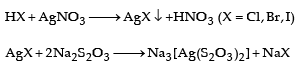*Multiple options can be correct
QUESTION: 12

With respect to graphite and diamond, which of the statement(s) given below is (are) correct ?

Solution:

(a) Diamond is harder than graphite.
(b) Graphite is good conductor of electricity as each carbon is attached to three C-atoms leaving one valency free, which is responsible for electrical conduction, while in diamond, all the four valencies of carbon are satisfied, hence insulator.
(c) Diamond is better thermal conductor than graphite.
Whereas electrical conduction is due to availability of free electrons; thermal conduction is due to transfer of thermal vibrations from atom to atom. A compact and precisely aligned crystal like diamond thus facilitates fast movement of heat.
(d) In graphite, C – C bond acquires double bond character, hence higher bond order than in diamond.

*Multiple options can be correct
QUESTION: 13

The correct statement(s) about O3 is(are)

Solution: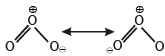Ozone is diamagnetic in nature (due to presence of paired electron) and both the O – O bond length are equal. It has a bent structure.

*Multiple options can be correct
QUESTION: 14

For the reaction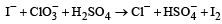Solution:

Balanced chemical equation is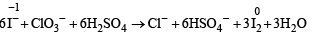*Multiple options can be correct
QUESTION: 15

The correct statement(s) for orthoboric acid is/are

Solution:

H3BO3 does not undergo self ionization. However, it acts as a weak acid in water (hence it is a weak electrolyte in water).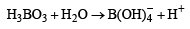Addition of cis-diols (e.g., ethylene glycol) to aqueous solution of orthoboric acid leads to complex formation, thus acidity of aqueous solution of orthoboric acid is increased.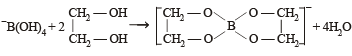*Multiple options can be correct
QUESTION: 16

The correct statement(s) regarding,

(i) HClO, (ii) HClO2, (iii) HClO3 and (iv) HClO4, is(are)

Solution: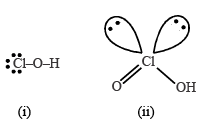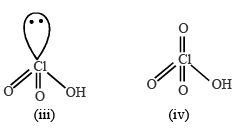Number of Cl = O bonds in (ii) and (iii) together is 3 Number of lone pairs on Cl in (ii) and (iii) together is 3 Hybridisation of Cl in all the four is sp3
Strongest acid is HClO4 (iv)

*Multiple options can be correct
QUESTION: 17

Under hydrolytic conditions, the compounds used for preparation of linear polymer and for chain termination, respectively, are

Solution:

(CH3)2SiCl2 form linear polymer on hydrolysis and (CH3)3SiCl is a chain terminator.

*Multiple options can be correct
QUESTION: 18

The crystalline form of borax has

Solution:

Structure of borax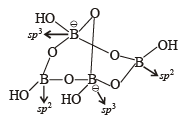Correct formula of borax is Na2[B4O5(OH)4].8H2O
(A) Borax has tetranuclear.  [B4O5(OH)4]2– unit
(B) Only two 'B' atom lie in same plane
(C) two Boron are sp2 and two are sp3 hybridised.
(D) one terminal hydroxide per boran atom

*Multiple options can be correct
QUESTION: 19

The nitrogen containing compound produced in the reaction of HNO3 with P4O10

Solution: